# Is Gold Going To Rise Or Fall? Gold Price Forecasting

Is Gold Going To Rise Or Fall? Gold Price Forecasting

#### Introduction & Interest of Research:

Gold is a unique asset class. As early as we first came into contact with economics, we were told that gold could be used as a general equivalent for trading other commodities. Gold has stable physical and chemical properties and static content in nature. Gold plays an important role in finance. After World War II, the Bretton Woods system directly determined that the dollar was directly linked to gold. This also established the hegemonic position of the US dollar.

At the same time, when the economic crisis and inflation are severe, people often choose to buy gold to resist inflation. This is the starting point of my interest. I want to study gold, a unique asset, to see if I can get new inspiration from the exchange data between the US dollar and gold. At the same time, I will use the ARIMA (4,0,2)-GARCH(1,1) model of time series to discuss and predict the price of gold. The coding environment used in this project is Jupyter Notebook with Python 3.9.

#### Data Source:

website: https://www.kaggle.com/code/andriimakarchuk/monthly-gold-prices-1990-2021-eda. In this data set, the price of gold in different periods with the currencies of 14 countries such as the US dollar, Japanese yen, euro, and RMB as the target is counted. As shown in the figure below: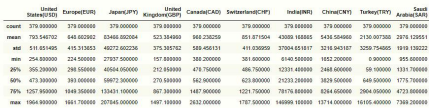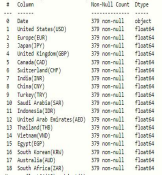However, in this project, we only use rate between gold rate against the United States dollar as our time series variable for the following reasons: First, as a common currency, theUS dollar is more familiar to us. Secondly, the trading volume of the US dollar is large, and the price game is more efficient than other currencies due to better liquidity. The most important point is that this paper aims to explore the significance of gold as an investment tool to investors. We use US dollars as the target, which is more in line with our daily habits. The following graphs summarize the exchange rate between gold and USD(or the gold price denoted by USD, we’ll call it “gold price” , denote as Gt )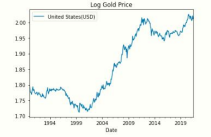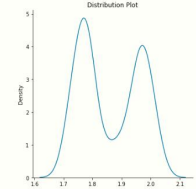The first graph shows the price change taking the logarithm and the second graph is the plot of the price distribution. The distribution is not Normal distribution since the price exhibits an increasing trend.

##### Before building our model, let’s do a quick calculation here as the “benchmark”. Let regress the logged gold price with time using OLS and see how the price behaves with time.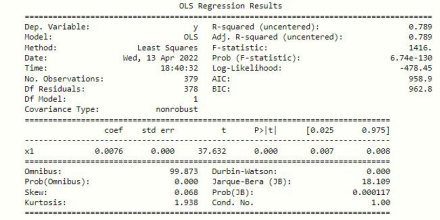By simply holding the gold, we got a monthly return of 0.0076 with a very significant p-value (around 9% annually multiplied by 12). However, the long-term trend we extracted is not enough to stabilize the residual term. It is a spurious regression. That is, the trend is likely to be a random trend. The goodness of fit, significance level and other indicators of spurious regression is perfect. However, because the residual sequence is a non-stationary sequence, this regression relationship can not truly reflect the equilibrium relationship between dependent variables and explanatory variables but is only a numerical coincidence. That’s why we want to apply the ARIMA model here.

#### ARIMA-GARCH MODEL:

(Step 1: First Difference)

We have prepared our data to use, let first look at auto-correlation and partial auto-correlation after we take logarithm: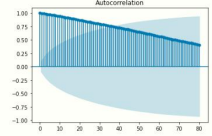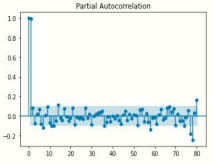These two graphs suggest the gold price has long-term memory, and more importantly, it

is not stationary. We need to make the series stationary before use. Furthermore, we denote the log priceasGt, the gold price at time t.

We took the first difference, and saw the result. The first graph is also the returns of the log price. Denote it as Rt, where Rt= (1-B)Gt.

After the first difference, it seems the series is stationary. To better verify the result, we use Augmented Dickey-Fuller test (ADF) to test the unit root in this sample. The output is: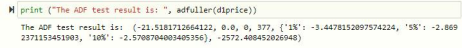P-value here is significantly less than 0.05. Thus, we reject that there is a unit root hypothesis.

After the first difference, the series is stationary. We also need to test if Rt is white noise. Because if Rt is white noise, then Gt is a random walk. It’s unpredictable. Here we use the

##### Ljung-Box statistic:

Under the significant level of 0.05, we reject the null hypothesis, take the alternative, and conclude that Rt is a stationary but not white noise process. We can apply the ARMAmodel to Rt or ARIMA to Gt, where d=1.

(Step 2: determine parameters)

After setting d=1, the next step is to get p and q. ACF, PACF, AIC, and BIC can be valid to determine p and q. Usually, by examining the PACF plot, we can observe AR(p), and by looking at the ACF plot, we can evaluate MA(q). However, recall the auto-correlation and partial auto-correlation. It’s not easy to observe such p and q. Then, I considered using AIC and BIC and plotted the heat map. By looping through all the possible combinations of p and q, the heat map is the plot as follows: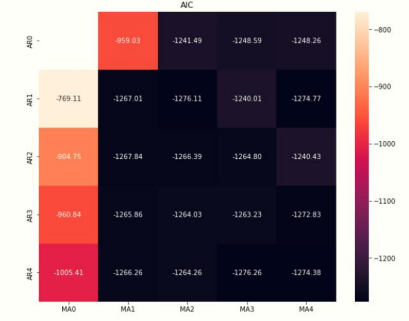This creates the first difficulty: we want a smaller AIC and small p and q. It means the

darker area on the heat map, the better result. However, the values are close. As long as p>1and q>1, the result would be fine. Then, I loop through the result using BIC. This gives me: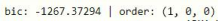##### Other models we can consider are: ARIMA(1,0,1), ARIMA(0,0,1).

I tried each one and eventually selected the model (4,0,2). This is because although other models have lower p and q, however, the coefficients are all not significant.

After determining the ARIMA(4,0,2) as the model, one thing is worth considering: dowe

Need a drift term? I ask this question because there seems to be a long-term upward trend for the gold price as it steadily increases. Moreover, a drift term will cause a significant change in the model since we need to centralize the parameters.

The expectation of returns is E(Rt)=0.00391. We performed a t-test on the sample andgot:

This means at the 5% level; we cannot reject the null. There’s no clear evidence to prove

the existence of the drift term.

##### (Step 3: Model diagnose)

After the model is established, we will diagnose the residual pattern. The residual is the part that the model cannot explain. The definition of residual is the difference between actual value and the predicted value. If the model is correctly identified, the relevant information contained in the sequence can be fully extracted. The residual should be Gaussian white noise with independent and identically distributed zero mean value. We useDubbin-Watson Statistic and qq-plot to test auto-correlation and the distribution of residuals.

The residual passed the auto-correlation test. However, it seems the

distribution is not normally distributed. We use the Shapiro-Wilk statistic to test the normality. The result shows the residual is indeed not normally distributed. A thin peak and thick

Tails are a common phenomenon in financial data; the probability of extreme values in the data is more significant than that in average distribution data. This will affect our previous

maximum likelihood estimation. In the deficiency and improvement of the model, we will further discuss this problem.

##### (Step 4: Model Forecasting)

Very excited, we are ready to make forecasts! I split the dataset into a training set andatesting set in this section. The training set includes the data from 1990 to 2018. The test set includes the last 40 observations. We employ the model above and make a forecast of the last 40 observations and compare them to actual values:

The green line is the forecast prices, and the yellow line is the original values, while the shaded area is 95% intervals of the prediction. However, the 95% interval includes all the actual values. This forecasting is honestly not satisfying at all. If we test the auto-correlationtest for new residuals, we will find it is not a white noise (with p-value = 2.69e-09!).

##### (Step 5: Model Deficiency and Improvement)

(a) The model is naive: The ARIMA model can only capture linear relationships inessence. It is established according to the internal relationship of the sequence, so it is challenging to add exogenous variables. However, the characteristics of financial series, especially in a large number of high-frequency transactions such as stocks and futures, are highly nonlinear. It is affected by various external factors and cannot be described by theARIMA model alone.

(b) Drift term: in the previous section, we exclude the drift term because the p-valueis0.08 > 0.05. However, our sample is monthly data and only includes 378 months. There likely exists a drift term that drives the price increase, as we observed in the graph. If a drift term exists, before estimating parameters, we need to centralize the returns: Rt =Rt- u; where u is the E(Rt).

(c) Volatility Process: The financial return series often has the phenomenon of fluctuationagglomeration. The price fluctuation of the stock market often has the characteristics of changing with time; that is, the fluctuation is sometimes relatively stable and sometimes highly intense. The return change often shows that it is continuously high or lowin a certainperiod. That is, its fluctuation has a positive correlation. Fluctuation agglomeration is a special heteroscedasticity phenomenon that will affect the estimation, Thus affecting theaccuracy of the model. This can be observed especially when a financial crisis happens. Wecan watch volatility using squared residuals, and this can also determine if we need the GARCH/ARCH model:

#### Final thoughts:

Is this the best prediction model? Of course not. Does this give a prediction of the future gold price? Probably not either. The lesson I learned here is that now I realize how hard it is to apply theory to reality. One thing is for sure: the annual return for holding gold will not be as high as 9% the following year. Using the final model, the actual return of somewhere around 4.4% is more reasonable. I checked the price today: \$1978. The return of recent one year is 4.1%. The result is quite close. This result also means it might be a good tool to hedge inflation (if the inflation rate is 2%).

Last but not least, I believe by using other currencies, we can undoubtedly find some pattern to hedge the risk. But I could not think of an excellent way to use that data. I trained my programming skills and time series knowledge further in this project. Thanks for reading!

#### Back To News

Is Gold Going To Rise Or Fall? Gold Price Forecasting